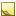﻿ Posit Class# Posit Class

3D pose estimation algorithm.Inheritance Hierarchy
SystemObject
Accord.Math.GeometryPosit

Namespace:  Accord.Math.Geometry
Assembly:  Accord.Math (in Accord.Math.dll) Version: 3.8.0Syntax
`public class Posit`

The Posit type exposes the following members.Constructors
NameDescriptionPosit
Initializes a new instance of the Posit class.
TopProperties
NameDescriptionFocalLength
Effective focal length of the camera used to capture the model.Model
Coordinates of the model points which pose should be estimated.
TopMethods
NameDescriptionEquals
Determines whether the specified object is equal to the current object.
(Inherited from Object.)EstimatePose
Estimate pose of a model from it's projected 2D coordinates.Finalize
Allows an object to try to free resources and perform other cleanup operations before it is reclaimed by garbage collection.
(Inherited from Object.)GetHashCode
Serves as the default hash function.
(Inherited from Object.)GetType
Gets the Type of the current instance.
(Inherited from Object.)MemberwiseClone
Creates a shallow copy of the current Object.
(Inherited from Object.)ToString
Returns a string that represents the current object.
(Inherited from Object.)
TopExtension Methods
NameDescriptionHasMethod
Checks whether an object implements a method with the given name.
(Defined by ExtensionMethods.)IsEqual
Compares two objects for equality, performing an elementwise comparison if the elements are vectors or matrices.
(Defined by Matrix.)To(Type)Overloaded.
Converts an object into another type, irrespective of whether the conversion can be done at compile time or not. This can be used to convert generic types to numeric types during runtime.
(Defined by ExtensionMethods.)ToTOverloaded.
Converts an object into another type, irrespective of whether the conversion can be done at compile time or not. This can be used to convert generic types to numeric types during runtime.
(Defined by ExtensionMethods.)
TopRemarks

The class implements an algorithm for 3D object's pose estimation from it's 2D coordinates obtained by perspective projection, when the object is described none coplanar points. The idea of the implemented math and algorithm is described in "Model-Based Object Pose in 25 Lines of Code" paper written by Daniel F. DeMenthon and Larry S. Davis (the implementation of the algorithm is almost 1 to 1 translation of the pseudo code given by the paper, so should be easy to follow).Note
At this point the implementation works only with models described by 4 points, which is the minimum number of points enough for 3D pose estimation.Note
The 4 model's point must not be coplanar, i.e. must not reside all within same planer. See CoplanarPosit for coplanar case.

Sample usage:

```// points of real object - model
Vector3[] positObject = new Vector3
{
new Vector3(  28,  28, -28 ),
new Vector3( -28,  28, -28 ),
new Vector3(  28, -28, -28 ),
new Vector3(  28,  28,  28 ),
};

// focal length of camera used to capture the object
float focalLength = 640; // depends on your camera or projection system

// initialize POSIT object
Posit posit = new Posit( positObject, focalLength );

// 2D points of te object - projection
Accord.Point[] projectedPoints = new Accord.Point
{
new Accord.Point(   -4,   29 ),
new Accord.Point( -180,   86 ),
new Accord.Point(   -5, -102 ),
new Accord.Point(   76,  137 ),
};

// estimate pose
Matrix3x3 rotationMatrix;
Vector3 translationVector;
posit.EstimatePose( projectedPoints,
out rotationMatrix, out translationVector );```See Also